# Evaluation of numerical simulation accuracy for two-ways mixed flow drip irrigation emitter based on CFD

Evaluation of numerical simulation accuracy for two-ways mixed flow drip irrigation emitter based on CFD

Lin Guo Dan Bai*Wen Zhou Xinduan Wang

Institute of Water Resources and Hydroelectric Engineering, Xi’an University of Technology, Xi’an, China

State Key Laboratory Base of Eco-Hydraulic Engineering in Arid Area, Xi’an University of Technology, Xi’an, China

School of Water Conservancy, North China University of Water Resources and Electric Power, Zhengzhou, China

Corresponding Author Email:
baidan@xaut.edu.cn
Page:
384-392
|
DOI:
https://doi.org/10.18280/ijht.350222
|
Accepted:
|
Published:
30 June 2017
| Citation

OPEN ACCESS

Abstract:

In order to evaluate the accuracy and applicability of the CFD numerical simulation method, we taken new two-ways mixed flow drip irrigation emitter as the research object, designed three kinds of emitter prototypes, and put forward the evaluation methods of the macroscopic flow rate index and the microscopic flow velocity index. According to the test results of flow rate and flow velocity by experiment and Particle Image Velocimetry (PIV) technology, analyzing the error of three kinds of wall function and seven classes physical models. The analysis results show that taking the macroscopic flow rate index as the evaluation standard, and numerical simulation accuracy of the enhanced wall function is higher and better. Comprehensive analysis of seven classes physical model by using the enhanced wall show that the average relative errors of the Standard k-ε model, RNG k-ε model, Standard k-ω model, SST k-ω model are smaller, followed by 2.16%, 1.47%, 1.84% and 1.90%, but RNG k-ε model has better simulation accuracy. Taking the microscopic flow velocity index as evaluation standard, the average relative errors are 3.42%, 2.68%, 2.34% and 1.81%, while the SST k-ω model has better simulation accuracy. Comprehensive evaluating two indexes, when the weight coefficient were taken at 0.5, respectively, the average relative error of SST k-ω model is calculated to be 1.85%, which is more suitable for the numerical simulation of emitter. The accuracy and applicability of various simulation methods are considered in the calculation of the flow rate and flow velocity, and the analysis of microscopic flow field.

Keywords:

drip irrigation emitter, numerical simulation, calculation accuracy, index, weight coefficient

1. Introduction

Structural design, hydraulic performance analysis and flow mechanism research of the emitter are based on the theory of hydromechanics and hydraulics . The experimental test [2-4], CFD numerical simulation [5-8], PIV flow field observation [9-11] and other methods are adopted to study the emitter. Whereas, for small size emitter and complex flow channel boundary, conventional experiment is difficult to meet the testing requirements, therefore, CFD has been widely used in the emitter research by domestic and foreign scholars, and experimental test as the basis of the study [12-14], is more used to verify the accuracy of numerical simulation .

The CFD numerical simulation technology is a kind of discrete calculation method [16-17], which can reduce the costs of research and development for emitter, shorten the cycle, and make up the disadvantages of experimental test . Yu L M , Wei Z Y , Jin W , Wei Q S [22-23], Wang W.E. , Yang P.L. , Ali-Mohammed  and other scholars had carried out in-depth research with CFD as the tool the hydraulic performance, flow mechanism, flow field distribution and the head loss  et al. However, different parameters setting, the choice of the physical model and the different processing methods of the wall function will affect the accuracy of the numerical simulation. Therefore, it is very important to analyze the applicability and accuracy of the numerical simulation. Li G Y et al.  found that the size of the grid cell was smaller than the minimum size of the 1/6, and further refinement the mesh had no significant impact on the simulation accuracy. Li Y K et al. [29-30] analyzed and contrasted the accuracy about realizable k-ε, Standard k-ε and LES physics model respectively, and found that the LES model was more accurate. Bai D et al.  had figured out that simulation values of realizable k-ε model consistent with the test values; Zhang J et al. [32-33] found that the flow rate calculation error was larger using laminar model The fluid classical theory did not apply to study on the fluid motion state of emitter, and the turbulence model was more suitable for simulation. Al-Muhammad et al.  used Standard k-ε and RNG k-ε model and compared with classical turbulence model, and found that the classical turbulence model was not suitable for flow analysis of low Reynolds number, however, simulation values of Standard k-ε and RNG k-ε closed to the experimental values. Palau et al.  believed the grid size was less than 0.19 mm, and the finer grid did not improve the accuracy of simulation.

Usually the evaluation method of numerical simulation accuracy for emitter is divided into two categories. The first is the evaluation method of macroscopic flow rate index, namely compared the relative error between the simulation values and test values of flow rate. The other is the evaluation method of microscopic flow velocity index, namely compared the relative error between the simulation values and test values of flow velocity of different points within channel. At present, the most scholars are based on macroscopic flow rate test, evaluate the accuracy of numerical simulation, but few scholars analyze and compare from the microscopic view. Therefore, based on the two-ways mixed flow drip irrigation emitter as the research object , comprehensively analyze two aspects of macroscopic and microscopic, establish the evaluation method of macroscopic flow rate index and microscopic flow velocity index, and different weight coefficient is calculated. Finally, comprehensively evaluate the applicability and accuracy of the numerical simulation method for CFD software. It is designed to provide method and idea for analyzing the numerical simulation accuracy for two-ways mixed flow drip irrigation emitter and improve the reliability of numerical simulation. This is to solve the main problem in this paper.

2. Structure of Emitter Flow Channel

2.1 Design of emitter

Figure 1 shows the emitter structure, in which the core components of energy dissipation are dividing water device and blocking water device, forming forward flow and backward flow, and producing mixed phenomenon, which effectively increase the local head loss of flow channel. It is essential to energy dissipation for drip irrigation emitter.

## 1.png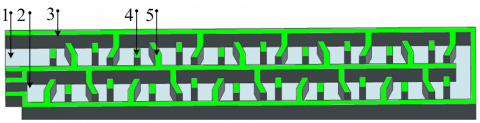Notes: 1. Inlet; 2. Outlet; 3. Flow channel side wall; 4. Dividing water device; 5. Blocking water device.

Figure 1. Structure of emitter

2.2 Geometric parameters and test prototype

Geometric parameters of the flow channel unit shown as Figure 2. The flow channel depth of emitter is 0.8 mm (D=0.8 mm).

## 2.png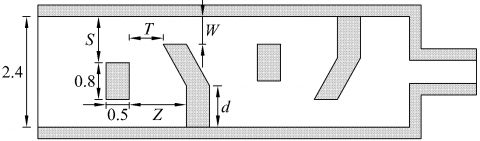Notes: 1. S is distance between dividing water device and flow channel side wall, mm; 2. T is distance between blocking water device tooth and dividing water device, mm; 3. W is distance between blocking water device and flow channel side wall, mm; 4. Z is maximal flow channel width of blocking water device and dividing water device, mm; 5. d is bottom pillar height of blocking water device, mm, same as below.

Figure 2. Geometry parameters of flow channel unit

The three kinds of the prototypes were designed, and focus on research the accuracy of the CFD simulation software. The flow channel depth of emitter is 0.8 mm (D=0.8 mm). The values of flow channel geometry parameters of emitters are shown in Table 1.

Table 1. Values of flow channel geometry parameters

 Prototypes Geometry parameters (mm) S T W Z d D 1 0.6 0.7 0.7 1.1 0.3 0.8 2 0.7 0.7 0.8 1.3 1.2 0.8 3 0.9 0.9 0.7 1.4 0.6 0.8

The prototypes of the experimental emitters are shown in Figure 3.

## 3.pngFigure 3. Prototypes of experimental emitters

3. CFD Numerical Simulation

3.1 Governing equations

The fluid in the emitter is water, so, it could be regarded as the typical viscous steady incompressible flow with the following governing equation.

Continuity equation:

$\frac{\partial u}{\partial x}+\frac{\partial v}{\partial y}+\frac{\partial w}{\partial z}=0$         (1)

Navier-Stokes equation:

$\frac{\partial(\rho u)}{\partial t}+\operatorname{div}(\rho u U)=\operatorname{div}(\mu \cdot \operatorname{grad} u)-\frac{\partial p}{\partial x}+F_{u}$    (2)

$\frac{\partial(\rho v)}{\partial t}+\operatorname{div}(\rho v U)=\operatorname{div}(\mu \cdot \operatorname{grad} v)-\frac{\partial p}{\partial y}+F_{v}$      (3)

$\frac{\partial(\rho w)}{\partial t}+\operatorname{div}(\rho w U)=\operatorname{div}(\mu \cdot \operatorname{grad} w)-\frac{\partial p}{\partial z}+F_{w}$    (4)

where U is the flow velocity, m/s; u, v, w is the velocity value on axes of x, y, and z, respectively, m/s; ρ is the water density, kg/m3; μ is the dynamical viscidity coefficient, N·s/m2; p is the fluid pressure, Pa; Fu, Fv, Fw is the value of mass force, respectively, N/m3, (considering only gravity), Fu=0, Fv=0, Fw=-ρg.

3.2 Grid unit settings

In this paper, the channel can be meshed with unstructured hybrid tetrahedron grids in order to get high quality grids. At the same time, it can automatically select the pyramid grid in the complex structure region, which makes the grid connection more smooth and real. The grid quality check in Gambit software is shown in Figure 4, Because of the large proportion of the column on the left side, it shows that the grid quality is very high, and the simulation results will not be distorted.Figure 4. Grid quality check

3.3 Wall function

In the process of numerical simulation, the wall function has a direct impact on the accuracy of the simulation results. Numerical simulation using different physical models is based on the choice of wall function. Therefore, the different wall function was adopted to improve the accuracy in the near wall of the flow channel, especially for the k-ε model, the wall function treatment has a more obvious effect on the accuracy of simulation. So we analyzed the accuracy of the standard wall function, the non-equilibrium wall function, and the enhanced wall function, respectively.

3.4 Physical model

The physical model of CFD software mainly includes S-A model, Standard k-ε model, RNG k-ε model, Realizable k-ε model, Standard k-ω model, SST k-ω model, RS model and so on. These results were compared with the experimental test results one by one.

4. Materials and Methods

4.1 Research route

The numerical simulation accuracy analysis method and the research route of the emitter are shown in Figure 5.

## 5.pngFigure 5. Analysis and research route

4.2 Emitter prototype processing

The emitter prototype consists of flow channel bottom plate and retention water cover plate. They were all made of organic glass which had flat and smooth surface. The high precision engraving technique was utilized to carve the channel with same ratio model in order to achieve ideal precision. Each structure was processed five prototypes, to reduce the machining error.

4.3 Experimental installation and PIV system

Experimental installation and Particle Image Velocimetry system is shown in Figure 6. Each structure was installed five prototypes, tested 3 times and each time lasted 5 mins.

## 6.png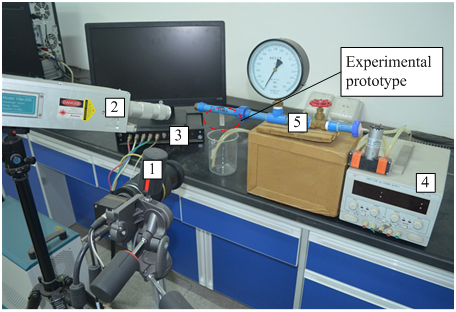Notes: 1. CCD camera; 2. Dual-pulse laser; 3. Synchronous controller; 4. DC Power; 5. Experimental installation.

Figure 6. Experimental installation and PIV system

4.4 Flow rate test

The relationship between flow rate and pressure is as follows under the different working pressure:

$q=k H^{x}$    (5)

Where q is the flow rate, L/h; k is the flow coefficient; H is the inlet pressure head, kPa; x is the flow index.

The flow rate values corresponding to different pressures are shown in Table 2.

Table 2. Flow rate testing results

 Prototype Pressure H (kPa) 50 90 130 170 210 250 1 2.311 3.008 3.561 4.022 4.427 4.803 2 2.296 2.941 3.458 3.909 4.304 4.679 3 2.246 2.879 3.391 3.817 4.188 4.549

Notes: Flow rate value is under the different pressure, q/(L/h).

5. Results and Analysis

5.1 Macroscopic flow rate index evaluation

5.1.1 Accuracy analysis of different wall function treatment

The Figure 7 shows the relationship between the simulation values of different wall functions and test values.

## 7a.png(a) Prototype 1

## 7b.png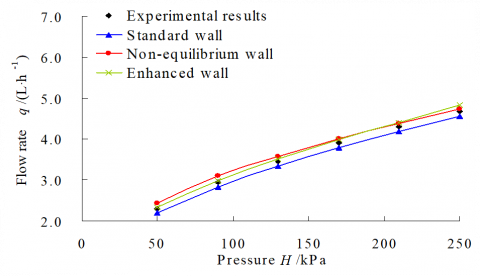(b) Prototype 2

## 7c.png(c) Prototype 3

Figure 7. Curve of q-h under the different wall function

The flow rate curves of different wall function treatment show that the average relative error of non-equilibrium wall function is 4.99%, especially in the 50~150 kPa, the average relative error is 7.01%. It is mainly caused by the fluctuation in lower pressure interval. The accuracy of the standard wall and the enhanced wall are better, the average relative errors are 2.57% and 2.16% respectively. Especially in 50~150 kPa, the average relative error of the enhanced wall treatment is only about 1.65%. By contrast, the enhanced wall is more accurate. It can reflect the real flow rate value of the emitter, especially for the low Reynolds number flow, which can well match the actual flow situation. The accurate choice of the wall function can eliminate the error caused by the simulation calculation. Therefore, we will use the enhanced wall function to compare accuracy of different physical models in follow-up study, so as to improve the veracity of different physical model calculation and the reliability of accuracy analysis.

5.1.2 Accuracy analysis of different physical models

The Figure 8 shows the relationship between the simulation values of different physical models and test values.

## 8.png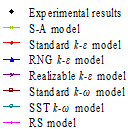## 8a.png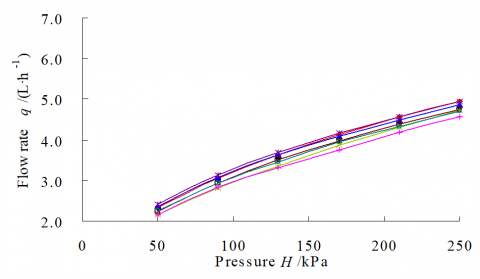(a) Prototype 1

## 8b.png(b) Prototype 2

## 8c.png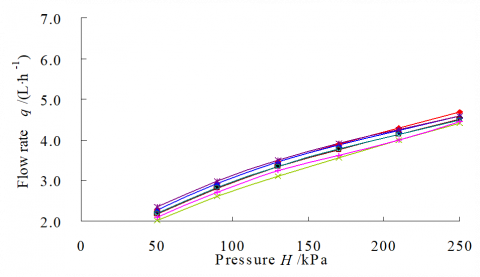(c) Prototype 3

Figure 8. Curve of q-h under the different physical model

The flow rate curves of different physical models show that the relative errors of S-A, Standard k-ε, RNG k-ε, Realizable k-ε, Standard k-ω, SST k-ω and RS models are 2.41%~7.01%, 2.03%~2.34%, 1.14%~1.73%, 1.28%~3.75%, 1.67%~2.16%, 1.47%~2.29% and 4.07%~6.01% respectively, and the average relative errors are 4.61%, 2.16%, 1.47%, 2.34%, 1.84%, 1.90% and 4.91% respectively. According to the results of relative error analysis, the average relative errors of S-A, Realizable k-ε and RS models are larger than other models. In addition, the deviation between the test values and simulation values of the single-equation S-A model is not obvious in 150~250 kPa, and the average relative error is 2.83%. But when the pressure is below 150 kPa, the average relative error is 6.39%. Furthermore, the S-A model is a single-equation model, which is insensitive to the error of the numerical simulation. The RS model is 7 equation turbulence models. It is more strictly and meticulously considering the anisotropic complex flow. But can see from the results that the RS model did not reach the expected accuracy, and the average relative error is 4.91%. At the same time, the Realizable k-ε and RS models are more complex computation models. The simulation results are unstable in the calculation process, and the calculation time is long and difficult to convergence. Therefore, these models are not suitable for simulating the emitter. However, the results of Standard k-ε model, RNG k-ε model, Standard k-ω model, SST k-ω model are in good agreement with the test values. Therefore, the four models were further analyzed. The Figure 9 shows the errors of the test values and simulation values of the four models.

## 9.png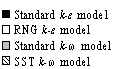## 9a.png(a) Prototype 1

## 9b.png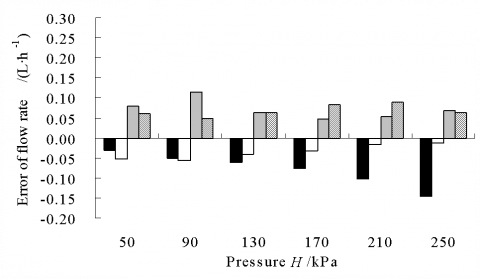(b) Prototype 2

## 9c.png(c) Prototype 3

Figure 9. Error of flow rate

The results show that the simulation results of the RNG k-ε model is close to test values, and the error is 0.013~0.067 L/h and the average relative error is 1.47%. The simulation accuracy of the Standard k-ω model and SST k-ω model is approximate, the average relative errors are 1.84% and 1.90% respectively, and the average relative error of the Standard k-ε model is large, which is about 2.16%.

5.2 Microscopic flow velocity index evaluation

The Figure 10 shows along with the flow direction from the inlet to the outlet of the flow channel to take the different position of the velocity point, and use PIV system to test the flow velocity at various points under different pressure. The Figure 11 shows the flow velocity comparison of the test values and simulation values of the four physical models under the pressure of 50~150 kPa.

## 10.pngFigure 10. The position of different velocity points

## 11a.png## 11b.png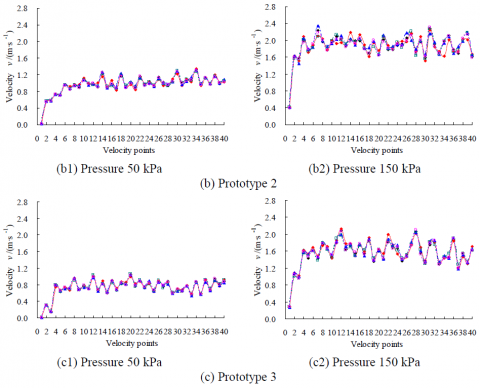Figure 11. Velocity of different points

It can be seen from the comparison of flow velocity in Figure 11 that the simulation values of each point are basically consistent with the test values. The relative error results show that the simulation values of SST k-ω model are closest to the test values, and the average relative error is 1.81%. The accuracy of the RNG k-ε model and the Standard k-ω model is approximate, and the average relative errors are 2.68% and 2.34% respectively. The average relative error of the Standard k-ε model is about 3.42%.

5.3 Comprehensive evaluation of numerical simulation accuracy

It is not difficult to see that the results of the two kinds of evaluation method with macroscopic flow rate index and microscopic flow velocity index are not consistent. This phenomenon is due to the applicability of the model. Therefore, when choosing the physical model, we need to combine the emphasis of the study, and introduce the weight coefficient. The ratio of weight coefficient is reasonably calculated, and evaluating the two indexes comprehensively. This paper focuses on establishment of a comprehensive evaluation method using two indexes of macroscopic and microscopic, and comprehensively compares CFD numerical simulation accuracy. It is designed to provide method and idea for analyzing the simulation accuracy. Therefore, the calculation method of weight coefficient based on the function driven principle was adopted (i.e. subjective weighting method). And considering the flow rate and flow velocity are equally important in this paper, so the weight coefficients are 0.5. We can get from the comprehensive calculation of two evaluation indexes that the average relative error of the Standard k-ε model is 2.79%, the average relative error of RNG k-ε model is 2.07%, the average relative error of the Standard k-ω model is 2.09% and the average relative error of SST k-ω model is 1.85%. To sum up, the SST k-ω model is more accurate and reasonable in flow rate calculation and flow field analysis.

6. Discussions

This paper puts forward evaluation methods of the macroscopic flow rate index and microscopic flow velocity index towards analysis of CFD simulation accuracy. We assume that the two indexes are equally important, so the weight coefficients of the two indexes are 0.5. But in the actual situation, it is necessary to consider the different calculation demand and the calculation expectation, and to choose the different weight coefficients. Starting from the subjective and objective perspective of statistics, the calculation method of weight coefficient is divided into calculation method based on the function driven principle (i.e. subjective weighting method), the difference drive principle (i.e. objective weighting method), and integrated calculation principle (i.e. subjective and objective integrated weighting method). So, weight coefficients are different using different calculation methods. The subjective weighting method is through the importance of different indexes to judge using subjective analysis and relative comparison, and then the value of weight coefficient is determined. If only consider the flow rate, it should be appropriately increased the weight of microscopic index, such as more emphasis on the analysis of internal flow velocity distribution and fluid motion state, which should be appropriately increased the weight of microscopic index. For the objective weighting method, it is not necessary to consider the importance of each index. The weight coefficient is calculated only by the statistical method such as the variation coefficient method, the multiple correlation coefficient method and the standard deviation method. Therefore, the weight calculation depended on the research object and the research emphasis of emitter, and considering different calculation requirement and calculation expectation.

7. Conclusions

Take new two-ways mixed flow drip irrigation emitter as the research object, and design three kinds of emitter prototype. Take the results of the flow rate test and flow velocity test as the basis, putting forward evaluation method of CFD simulation accuracy the including macroscopic flow rate index and microscopic flow velocity index. The simulation accuracy and applicability of three kinds of wall function and seven classes physical models are analyzed as following:

1) The average relative error between the test values and the simulation values of non-equilibrium wall treatment method is 4.99%, especially in the lower pressure interval of 50~150 kPa, which is about 7.01%. The simulation results of the standard wall treatment method and enhanced wall treatment method is more accurate, especially in the lower pressure interval, the simulation values of enhanced wall treatment method is very close to test values. The average relative error is only 1.65%, which can accurately reflect the real the flow rate values of emitter.

2) Taking the macroscopic flow rate index as the evaluation standard, the simulation accuracy of the S-A model and RS model in the seven classes physical models are poor, and the average relative errors are 4.61% and 4.91%, respectively. The simulation accuracy of k-ε model and k-ω model are better. The average relative errors between the test values and simulation values of Standard k-ε model, RNG k-ε model, Standard k-ω model, SST k-ω model are 2.16%, 1.47%, 1.84% and 1.90%. The calculation accuracy of RNG k-ε model is best.

3) Taking the microscopic flow velocity index as the evaluation standard. The average relative errors between the test values and simulation values of Standard k-ε model, RNG k-ε model, Standard k-ω model, SST k-ω model are 3.42%, 2.68%, 2.34% and 1.81%. The calculation accuracy of SST k-ω model is best.

4) Evaluating and contrasting two indexes comprehensively, given the weight coefficients of two indexes are 0.5, respectively. So, the average relative error of SST k-ω model is 1.85%, which is more accurate and reasonable in the calculation of flow rate and flow velocity, and the analysis of microscopic flow regime.

Acknowledgments

Author is grateful for financial support from the National Natural Science Fund of China (No. 51279156, 41571222) and the Specialized Research Fund for the Doctoral Program of Higher Education of China (No. 20116118110010). The experiment site was provided by the State Key Laboratory Base of Eco-Hydraulic Engineering in Arid Area, Xi’an University of Technology.

Nomenclature
 Fu value of mass force on axes of x, N/m3 Fv value of mass force on axes of y, N/m3 Fw value of mass force on axes of z, N/m3 H inlet pressure head, kPa k flow coefficient p fluid pressure, Pa q flow rate, L/h u velocity value on axes of x, m/s U flow velocity, m/s v velocity value on axes of y, m/s w velocity value on axes of z, m/s Greek symbols μ dynamical viscidity coefficient, N·s/m2 ρ water density, kg/m3
References

 Vekariya P.B., Subbaiah R., Mashru H.H. (2011). Hydraulics of microtube emitters: a dimensional analysis approach, Irrigation Science, Vol. 29, No. 4, pp. 341-350. DOI: 10.1007/s00271-010-0240-6

 Tayel M., Lightfoot D., Mansour H. (2012). Effects of drip irrigation circuit design and lateral line lengths: I-on pressure and friction loss, Agricultural Sciences, Vol. 3, No. 3, pp. 392-399. DOI: 10.4236/as.2012.33046

 De Jesus S. W., Sinobas L.R., Sánchez R., Botrel T.A., Coelho R.D. (2014). Prototype emitter for use in subsurface drip irrigation: manufacturing, hydraulic evaluation and experimental analyses, Biosystems Engineering, Vol. 128, pp. 41-51. DOI: 10.1016/j.biosystemseng.2014.09.011

 Li Z.Q., Ma J. (2012). Experiment on flow pattern in labyrinth emitter, Transactions of the Chinese Society of Agricultural Engineering, Vol. 28, No. 1, pp. 82-86. DOI: 10.3969/j.issn.1002-6819.2012.01.016

 Rafiee S.E., Sadeghiazad M.M. (2016). Three-dimensional CFD simulation of fluid flow inside a vortex tube on basis of an experimental model- the optimization of vortex chamber radius, International Journal of Heat and Technology, Vol. 34, No. 2, pp. 236-244. DOI: 10.18280/ijht.340212

 Lasbet Y., Aidaoui L., Loubar K. (2016). Effects of the geometry scale on the behaviour of the local physical process of the velocity field in the laminar flow, International Journal of Heat and Technology, Vol. 34, No. 3, pp. 439-445. DOI: 10.18280/ijht.340313

 Boukhalkhal A.L., Lasbet Y., Makhlouf M., Loubar K. (2017). Numerical study of the chaotic flow in three-dimensional open geometry and its effect on the both fluid mixing and heat performances, International Journal of Heat and Technology, Vol. 35, No. 1, pp. 1-10. DOI: 10.18280/ijht.350101

 Shukla A.K., Anupam D. (2017). Flow and thermal characteristics of jet impingement: comprehensive review, International Journal of Heat and Technology, Vol. 35, No. 1, pp. 153-166. DOI: 10.18280/ijht.350121

 Caruso G., Cristofano L., Nobili M., Romano G.P. (2016). Experimental investigation on free surface vortices driven by tangential inlets, International Journal of Heat and Technology, Vol. 34, No. 4, pp. 653-662. DOI: 10.18280/ijht.340415

 Corvaro F., Nardini G., Paroncini M., Vitali R. (2015). Piv and numerical analysis of natural convective heat transfer and fluid flow in a square cavity with two vertical obstacles, International Journal of Heat and Technology, Vol. 33, No. 2, pp. 51-56. DOI: 10.18280/ijht.330208

 Silva G., Leal N., Semiao V. (2008). Micro-PIV and CFD characterization of flows in a microchannel: velocity profiles, surface roughness and Poiseuille numbers, International Journal of Heat and Fluid Flow, Vol. 29, No. 4, pp. 1211-1220. DOI: 10.1016/j.ijheatfluidflow.2008.03.013

 Dayal P.A.S., Raju G.S.N., Mishra S. (2016). Pattern synthesis using accelerated particle swarm optimization, Modelling, Measurement and Control A, Vol. 89, No. 1, pp. 58-76.

 Mukhopadhyay N., Mondal P. (2016). Optimization of combined conductive and convective heat transfer model of a cold storage using Taguchi S/N ratio analysis, Modelling, Measurement and Control B, Vol. 85, No. 1, pp. 63-78.

 Tian X.H. (2016). Deployment algorithm using fluid dynamics for agriculture sensor networks, Modelling, Measurement and Control B, Vol. 85, No. 1, pp. 175-185.

 Aydin M.C. (2012). CFD simulation of free-surface flow over triangular labyrinth side weir, Advances in Engineering Software, Vol. 45, No. 1, pp. 159-166. DOI: 10.1016/j.advengsoft.2011.09.006

 Zeiny E., Farhadi M., Sedighi K. (2017). Numerical investigation of the simultaneous influence of swirling flow and obstacles on plate in impinging jet, International Journal of Heat and Technology, Vol. 35, No. 1, pp. 59-66. DOI: 10.18280/ijht.350108

 Triveni M.K., Panua R. (2017). Numerical analysis of natural convection in a triangular cavity with different configurations of hot wall, International Journal of Heat and Technology, Vol. 35, No. 1, pp. 11-18. DOI: 10.18280/ijht.350102

 Wang F.J., Wang W.E. (2006). Research progress in analysis of flow passage in irrigation emitters using computational fluid dynamics techniques, Transactions of the Chinese Society of Agricultural Engineering, Vol. 22, No. 7, pp. 188-192.

 Yu L.M., Zou X.Y., Tan H., Yan W.G., Chen L.Z., Xiong Z.W. (2016). 3D numerical simulation of water and sediment flow in hydrocyclone based on coupled CFD-DEM, Transactions of the Chinese Society for Agricultural Machinery, Vol. 47, No. 1, pp. 126-132. DOI: 10.6041/j.issn.1000-1298.2016.01.017

 Wei Z.Y., Tang Y.P., Zhao W.H., Lu B.H. (2009). Visualized experimental analysis of water-sand flow behaviors for labyrinth emitters, Journal of Mechanical Engineering, Vol. 45, No. 8, pp. 89-94. DOI: 10.3901/jme.2009.08.089

 Jin W., Zhang H.Y. (2010). Numerical Simulation approaches and experiment on micro-scales flow field, Transactions of the Chinese Society for Agricultural Machinery, Vol. 41, No. 3, pp. 67-71. DOI: 10.3969/j.issn.1000-1298.2010.03.014

 Wei Q.S., Lu G., Liu J., Shi Y.S., Dong W.C., Huang S.H. (2008). Evaluations of emitter clogging in drip irrigation by two-phase flow simulations and laboratory experiments, Computers and Electronics in Agriculture, Vol. 63, No. 2, pp. 294-303. DOI: 10.1016/j.compag.2008.03.008

 Wei Q.S., Shi Y.S., Dong W.C., Liu J., Huang S.H. (2006). Study on hydraulic performance of drip emitters by computational fluid dynamics, Agricultural Water Management, Vol. 84, No. 1-2, pp. 130-136. DOI: 10.1016/j.agwat.2006.01.016

 Wang W.E., Wang F.J. (2010). Numerical simulation of unsteady flow in labyrinth emitters of drip irrigation system, Journal of Hydraulic Engineering, Vol. 41, No. 3, pp. 332-337. DOI: 10.13243/j.cnki.slxb.2010.03.016

 Zhang Z.L.L., Yang P.L., Ren S.M., Wu D. (2013). Numerical simulation and optimization of micro-irrigation flow regulators based on FSI, Irrigation and Drainage, Vol. 62, No. 5, pp. 624-639. DOI: 10.1002/ird.1772

 Ali A.A.M. (2013). Anti-clogging drip irrigation emitter design innovation, European International Journal of Science and Technology, Vol. 2, No. 8, pp. 154-164.

 Yan H.J., Ou Y.J., Nakano K., Xu C.B. (2009). Numerical and experimental investigations on internal flow characteristic in the impact sprinkler, Irrigation and Drainage Systems, Vol. 23, No. 1, pp. 11-23. DOI: 10.1007/s10795-009-9061-2

 Li G.Y., Wang J.D., Alam M., Zhao Y.F. (2006). Influence of geometrical parameters of labyrinth flow path of drip emitters on hydraulic and anti-clogging performance, Transactions of the ASABE, Vol. 49, No. 3, pp. 637-643.

 Li Y.K., Liu H.S., Yang P.L., Wu D. (2013). Analysis of tracing ability of different sized particles in drip irrigation emitters with computational fluid dynamics, Irrigation and Drainage, Vol. 62, No. 3, pp. 340-351. DOI: 10.1002/ird.1737

 Wu D., Li Y.K., Liu H.S., Yang P.L., Sun H.S., Liu Y.Z. (2013). Simulation of the flow characteristics of a drip irrigation emitter with large eddy methods, Mathematical and Computer Modelling, Vol. 58, No. 3, pp. 497-506. DOI: 10.1016/j.mcm.2011.10.074

 Tian J.Y., Bai D., Yu F.L., Wang X.D., Guo L. (2014). Numerical simulation of hydraulic performance on bidirectional flow channel of drip irrigation emitter using Fluent, Transactions of the Chinese Society of Agricultural Engineering, Vol. 30, No. 20, pp. 65-71. DOI: 10.3969/j.issn.1002-6819.2014.20.009

 Zhang J., Zhao W.H., Wei Z.Y., Tang Y.P., Lu B.H. (2007). Numerical and experimental study on hydraulic performance of emitters with arc labyrinth channels, Computers and Electronics in Agriculture, Vol. 56, No. 2, pp. 120-129. DOI: 10.1016/j.compag.2007.01.007

 Zhang J., Zhao W.H., Tang Y.P., Wei Z.Y., Lu B.H. (2007). Numerical investigation of the clogging mechanism in labyrinth channel of the emitter, International Journal for Numerical Methods in Engineering, Vol. 70, No. 13, pp. 1598-1612. DOI: 10.1002/nme.1935

 Al-Muhammad J., Tomas S., Ait-Mouheb N., Amielh M., Anselmet F. (2014). μ-PIV characterization of the flow in a milli-labyrinth-channel used in micro-irrigation, Preceeding of 17th International Symposium on Applications of Laser Techniques to Fluid Mechanics, Lisbon, Portugal, pp. 1-15.

 Palau S.G., Arviza V.J., Bralts V.F. (2004). Hydraulic flow behaviour through an in-line emitter labyrinth using CFD techniques, Proceeding of 2004 ASAE/CSAE Annual International Meeting, Ottawa, Canada, pp. 3127-3135.

 Guo L., Bai D., Wang X.D., He J., Zhou W., Cheng P. (2016). Hydraulic performance and energy dissipation effect of two-ways mixed flow emitter in drip irrigation, Transactions of the Chinese Society of Agricultural Engineering, Vol. 32, No. 17, pp. 77-82. DOI: 10.11975/j.issn.1002-6819.2016.17.011